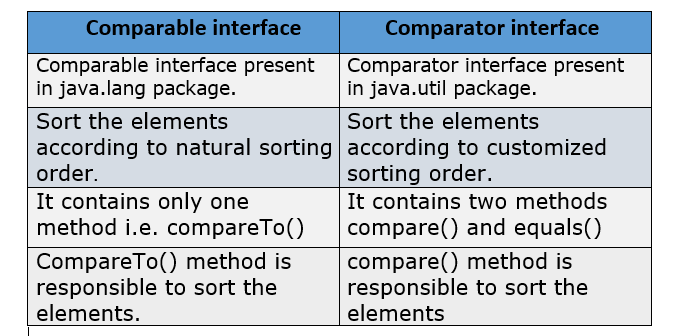Comparable in Java is an interface which is present in java.lang package. A comparable object is used to comparing itself with other objects. The objects of classes that implement the Comparable interface can be ordered. In other words, classes that implement the Comparable interface contain an object that can be compared in some meaningful manner. It contains only one method named compareTo(Object obj).

public int compareTo(Object obj): The compareTo(Object obj) method is used to compare the current object with the specified object.
1) It returns a positive integer value if the current object is less than the specified object.
2) It returns a negative integer value if the current object is greater than the specified object.
3) It returns zero if the current object is equal to the specified object.

### Example to demonstrate compareTo(Object obj) method:

``````
class compareToExample{

public static void main(String args[]){
System.out.println("A".compareTo("B"));
System.out.println("B".compareTo("A"));
System.out.println("A".compareTo("A"));
}
}
``````

### When we should go for Comparable interface?

If we want to sort the elements according to default natural sorting order than we should go for Comparable interface. If we go for default natural sorting order than object should be homogeneous and comparable. An object is said to be comparable if its corresponding class implement the Comparable interface. All the String and Wrapper classes by default implement the Comparable interface. Therefore if you want to sort String element than you don't need to implement Comparable interface.

## Java Comparable Example:

Suppose you have a class Employee and you want to sort employee id on the basis of natural sorting order which is ascending order for numbers and alphabetical order for String then you can implement Comparable interface with the Employee class and override the CompareTo(Object obj) method of Comparable interface. We can sort the element of the String object, wrapper class object, and user-defined class object. Here we have discussed the user-defined class object.

``````
import java.util.*;
class Employee implements Comparable<Employee>{
int eid;
String name;
int age;

//constructor for initialize the value
Employee(int eid,String name,int age){
this.eid=eid;
this.name=name;
this.age=age;
}

//compareTo() method.
public int compareTo(Employee emp){
if(eid==emp.eid)
return 0;
else if(eid>emp.eid)
return +1;
else
return -1;
}
}

public class ComparableDemo{
public static void main(String args[]){

ArrayList<Employee> al=new ArrayList<Employee>();

//call sort() method of collection interface
Collections.sort(al);

//for each loop to print the employee details
for(Employee emp:al){
System.out.println(emp.eid+" "+emp.name+" "+emp.age);
}
}
}  ``````

Output:

## Comparators in Java

Comparators in Java is an interface which is present in java.util package. Sometimes we do not satisfy with default natural sorting order then we should go for Customized sorting order. For customized sorting order we need to specify a Comparator when you construct a tree set. The comparator interface contains two methods:

1. public int compare(Object obj1, Object obj2): This method is used to compare the first object with the second object. It is similar to compareTo(Object obj) method of Comparable interface. This method return positive integer value if obj1 is greater then obj2, and returns negative integer value if the obj1 is less than the obj2. If the obj1 and obj2 are equals then it returns 0. A ClassCastException has occurred if the types of the objects are not compatible for comparison.

Read: What is an Exception and how to handle it in Java?

2. public boolean equals(Object obj): This method is used to compare the current object with the specified object. Overriding equals() method is optional because it is already available in every Java class from Object class through inheritance.

## Java Comparator Example:

In this example, we are going to show you how we use a comparator for customized sorting order. Here we are trying to insert integer value in the tree set according to descending order(own customized order). Let's understand this with a simple example
``````

import java.util.*;
class ComparatorDemo{
public static void main(String args[]){
TreeSet tset = new TreeSet(new MyComparator());

//displaying custom sorted order
System.out.println("Custom sorted order is: " +tset);
}
}

//our own class implements comparator interface
class MyComparator implements Comparator{
public int compare(Object obj1, Object obj2){

//typecast to an integer.
Integer I1 = (Integer)obj1;
Integer I2 = (Integer)obj2;
if(I1<I2)
return +1;
else if(I1>I2)
return -1;
else
return 0;
}
}``````

Output:

## Comparision table Comparable vs Comparator in JavaYou may also like these related posts:
Comparable vs Comparator in Java with ExampleReviewed by Prashant Srivastava on March 09, 2019 Rating: 5